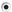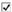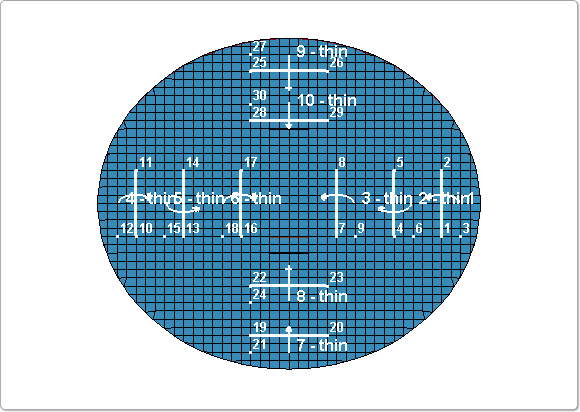# Tutorial 7: Page 4

Home » Knowledge Base » LS-Prepost » Tutorials » Extended Tutorials » Tutorial 7 » Tutorial 7: Page 4

• 4. Create Thin fold definitions #2-9
• N1=4, N2=5, Active=6, selectDown
• click Apply

• N1=7, N2=8, Active=9, selectUp
• click Apply

• N1=10, N2=11, Active=12, selectUp
• click Apply

• N1=13, N2=14, Active=15, selectDown
• click Apply

• N1=16, N2=17, Active=18, selectUp
• click Apply

• N1=19, N2=20, Active=21, selectDown
• click Apply

• N1=22, N2=23, Active=24, selectUp
• click Apply

• N1=25, N2=26, Active=27, selectDown
• click Apply

• N1=28, N2=29, Active=30, selectUp
• activateIgnore
• click Apply (see figure 7)

•• Figure 7